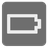Completely Empty
Simple+
English RU

You need to figure if a wellfounded and wellsized iterable is completely empty.

An iterable x0 is wellfounded if there is no infinite sequence

`x1,x2,x3...`
such that
`... in x3 in x2 in x1 in x0`
(where
`in`
is meant iteratively,
`x(n+1)`
will be encountered while iterating through
`xn`
).

A wellfounded iterable is wellsized if it has only finitely many iterable elements, and all of them are wellsized.

A wellfounded iterable is completely empty when all its elements are completely empty.

Some consequences of the above definitions:

• any empty iterable is completely empty
• a non-iterable is never completely empty
• the only wellfounded string is
`''`
, and it is completely empty
• bytes, and (possibly nested) tuples/frozensets of them are always wellfounded and wellsized
• `{'': 'Nonempty'}`
is a wellfounded and completely empty iterable
• after
`c=[];c.append(c)`
, c is a non-wellfounded iterable
• `itertools.repeat(())`
is wellfounded but not wellsized
• `itertools.repeat(5)`
is wellfounded and wellsized

Input: A wellfounded and wellsized iterable.

Output: A bool.

Example:

```completely_empty([]) == True
completely_empty() == False
completely_empty([[]]) == True
completely_empty([[],[]]) == True
completely_empty([[[]]]) == True
completely_empty(['']) == True
completely_empty([[],[{'':'No WAY'}]]) == True
```